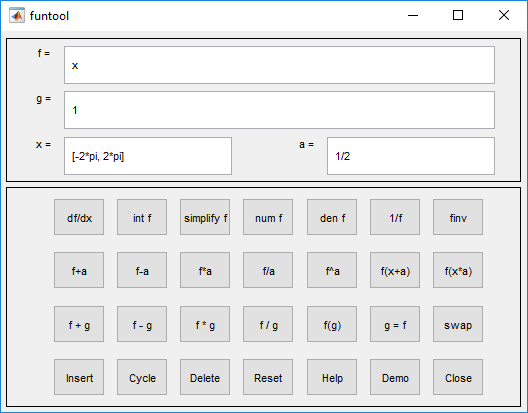Documentation

# funtool

Function calculator

## Syntax

``funtool``

## Description

````funtool` is a visual function calculator that manipulates and displays functions of one variable. At the click of a button, for example, `funtool` draws a graph representing the sum, product, difference, or ratio of two functions that you specify. `funtool` includes a function memory that allows you to store functions for later retrieval.At startup, `funtool` displays graphs of a pair of functions, `f(x) = x` and `g(x) = 1`. The graphs plot the functions over the domain `[-2*pi, 2*pi]`. `funtool` also displays a control panel that lets you save, retrieve, redefine, combine, and transform `f` and `g`.The top of the control panel contains a group of editable text fields. f= Displays a symbolic expression representing `f`. Edit this field to redefine `f`. g= Displays a symbolic expression representing `g`. Edit this field to redefine `g`. x= Displays the domain used to plot `f` and `g`. Edit this field to specify a different domain. a= Displays a constant factor used to modify `f` (see button descriptions in the next section). Edit this field to change the value of the constant factor. `funtool` redraws `f` and `g` to reflect any changes you make to the contents of the control panel's text fields.The bottom part of the control panel contains an array of buttons that transform `f` and perform other operations.The first row of control buttons replaces `f` with various transformations of `f`. df/dx Derivative of `f` int f Integral of `f` simplify f Simplified form of `f`, if possible num f Numerator of `f` den f Denominator of `f` 1/f Reciprocal of `f` finv Inverse of `f`The operators int f and finv can fail if the corresponding symbolic expressions do not exist in closed form.The second row of buttons translates and scales `f` and the domain of `f` by a constant factor. To specify the factor, enter its value in the field labeled a= on the calculator control panel. The operations aref+aReplaces `f(x)` by ```f(x) + a```.f-aReplaces `f(x)` by ```f(x) - a```.f*aReplaces `f(x)` by ```f(x) * a```.f/aReplaces `f(x)` by ```f(x) / a```.f^aReplaces `f(x)` by ```f(x) ^ a```.f(x+a)Replaces `f(x)` by ```f(x + a)```.f(x*a)Replaces `f(x)` by ```f(x * a)```.The first four buttons of the third row replace `f` with a combination of `f` and `g`.f+gReplaces `f(x)` by ```f(x) + g(x)```.f-gReplaces `f(x)` by `f(x)-g(x)`.f*gReplaces `f(x)` by ```f(x) * g(x)```.f/gReplaces `f(x)` by ```f(x) / g(x)```.The remaining buttons on the third row interchange `f` and `g`.g=fReplaces `g` with `f`.swapReplaces `f` with `g` and `g` with `f`.The first three buttons in the fourth row allow you to store and retrieve functions from the calculator's function memory.Insert Adds `f` to the end of the list of stored functions.Cycle Replaces `f` with the next item on the function list.Delete Deletes `f` from the list of stored functions.The other four buttons on the fourth row perform miscellaneous functions:ResetResets the calculator to its initial state.Help Displays the online help for the calculator.DemoRuns a short demo of the calculator.CloseCloses the calculator's windows. ```

## See Also

#### Mathematical Modeling with Symbolic Math Toolbox

Get examples and videos